# 7th Grade - Area of Composite Figures

## Introduction

• Composite figures are obtained by combining two or more geometric figures.
• For example, the composite figure shown below is obtained by combining a rectangle and a triangle.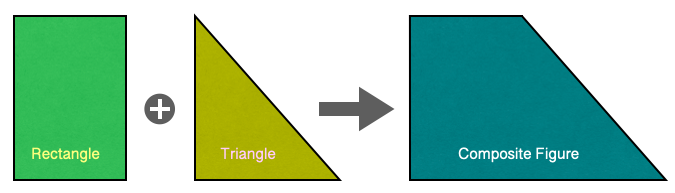## Area of Composite Figures

• The area of a composite figure is the surface measurement or square units it covers.
• The area of a composite figure can be calculated in the steps mentioned below.
1. Decomposing (breaking apart) the composite figure into basic geometric shapes such as rectangles, squares, triangles, circles, etc.
2. Adding/subtracting the area of each shape
• The area of some of the standard shapes is mentioned below.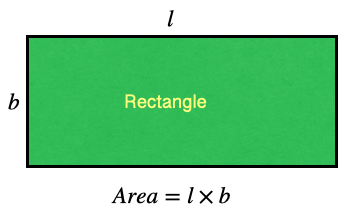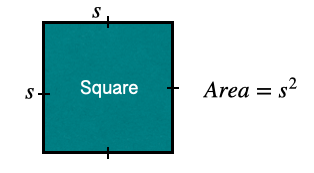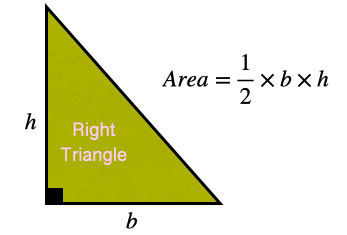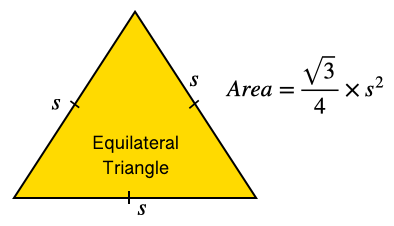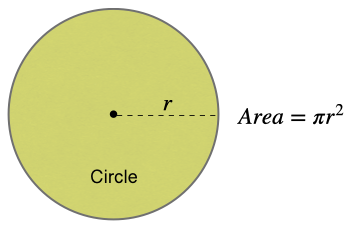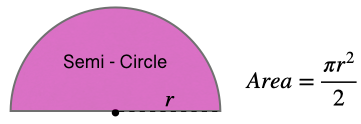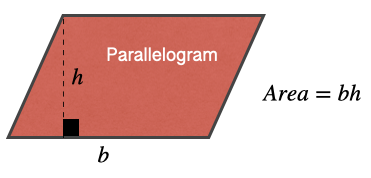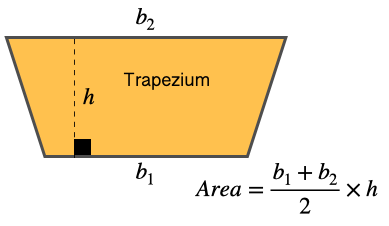## Perimeter of Composite Figures

• The perimeter of a composite figure is the measure of the length of its boundary.
• For example, the perimeter of the composite figure shown below is the sum of the three sides of a rectangle and the circumference of the semi-circle.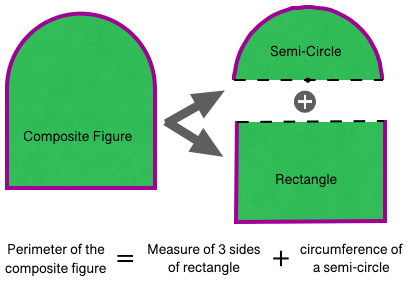## Solved Examples

Question 1: Find the area of the composite figure shown below.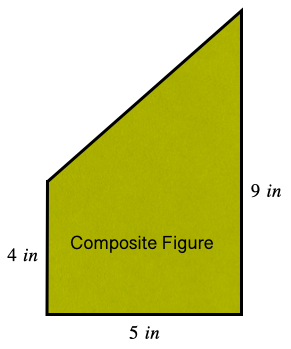Solution: The given composite figure can be represented as the combination of a rectangle and a triangle, as shown below.